• Python格式化日期时间的函数为datetime.datetime.strftime() 由字符串转为日期型的函数为：datetime.datetime.strptime() %a Abbreviated weekday name %A Full weekday name %b Abbreviated month name ...

Python格式化日期时间的函数为datetime.datetime.strftime()
由字符串转为日期型的函数为：datetime.datetime.strptime()

%a Abbreviated weekday name
%A Full weekday name
%b Abbreviated month name
%B Full month name
%c Date and time representation appropriate for locale
%d Day of month as decimal number (01 - 31)
%H Hour in 24-hour format (00 - 23)
%I Hour in 12-hour format (01 - 12)
%j Day of year as decimal number (001 - 366)
%m Month as decimal number (01 - 12)
%M Minute as decimal number (00 - 59)
%p Current locale's A.M./P.M. indicator for 12-hour clock
%S Second as decimal number (00 - 59)
%U Week of year as decimal number, with Sunday as first day of week (00 - 51)
%w Weekday as decimal number (0 - 6; Sunday is 0)
%W Week of year as decimal number, with Monday as first day of week (00 - 51)
%x Date representation for current locale
%X Time representation for current locale
%y Year without century, as decimal number (00 - 99)
%Y Year with century, as decimal number
%z, %Z Time-zone name or abbreviation; no characters if time zone is unknown
%% Percent sign


ebay中时间格式为‘Sep-21-09 16:34’
则通过下面代码将这个字符串转换成datetime

print(datetime.datetime.strptime('Sep-21-09 16:34','%b-%d-%y %H:%M'))
#2009, 9, 21, 16, 34

datetime转换成字符串

print(datetime.datetime.strptime('Sep-21-09 16:34','%b-%d-%y %H:%M'))
#2009, 9, 21, 16, 34

转载于:https://www.cnblogs.com/LiGoHi/p/7346120.html
展开全文• 今天帮朋友写自动化脚本，又需要用格式化日期，又忘记怎么写了，还是写到自己博客里面，方便日后需要的时候看一眼吧。So，临时加一篇 Python 的文章。 1、Python的time模块 import time print(time.time()) #...
作者新建了QQ群：460430320，供大家交流测试心得（培训机构勿进）。另外，还会不定期上传测试资料，也欢迎您共享测试资料。

今天帮朋友写自动化脚本，又需要用格式化日期，又忘记怎么写了，还是写到自己博客里面，方便日后需要的时候看一眼吧。So，临时加一篇 Python 的文章。

1、Python的time模块

import time

print(time.time())  #输出的是时间戳
print(time.localtime(time.time()))   #作用是格式化时间戳为本地的时间
# 最后用time.strftime()方法，把刚才的一大串信息格式化成我们想要的东西

print(time.strftime('%Y-%m-%d',time.localtime(time.time()))) 

运行结果：

C:\Python36\python.exe E:/python/test1/day1/test10.py
1508229738.234413
time.struct_time(tm_year=2017, tm_mon=10, tm_mday=17, tm_hour=16, tm_min=42, tm_sec=18, tm_wday=1, tm_yday=290, tm_isdst=0)
2017-10-17

2、time.strftime()方法参数
time.strftime里面有很多参数，可以让你能够更随意的输出自己想要的东西：
下面是time.strftime的参数：
strftime(format[, tuple]) -> string
将指定的struct_time(默认为当前时间)，根据指定的格式化字符串输出
python中时间日期格式化符号：
%y 两位数的年份表示（00-99）
%Y 四位数的年份表示（000-9999）
%m 月份（01-12）
%d 月内中的一天（0-31）
%H 24小时制小时数（0-23）
%I 12小时制小时数（01-12）
%M 分钟数（00=59）
%S 秒（00-59）
%a 本地简化星期名称
%A 本地完整星期名称
%b 本地简化的月份名称
%B 本地完整的月份名称
%c 本地相应的日期表示和时间表示
%j 年内的一天（001-366）
%p 本地A.M.或P.M.的等价符
%U 一年中的星期数（00-53）星期天为星期的开始
%w 星期（0-6），星期天为星期的开始
%W 一年中的星期数（00-53）星期一为星期的开始
%x 本地相应的日期表示
%X 本地相应的时间表示
%Z 当前时区的名称
%% %号本身

3、其它格式输出

（1）输出年月日时分秒

print(time.strftime('%Y-%m-%d %H:%M:%S',time.localtime(time.time())))

2017-10-17 16:53:30

（2）输出年月日，以斜杠分隔

print(time.strftime('%Y/%m/%d %H:%M:%S',time.localtime(time.time())))

2017/10/17 16:54:46

（3）显示年月日时分秒，中间无分隔，常用作保存日志的文件名

print(time.strftime('%Y%m%d%H%M%S',time.localtime(time.time())))

20171017165540


展开全文• python格式化输出日期
python time模块格式化输出时间
import time//导入time模块库
print time.strftime('%Y-%m-%d')//调用time库的strftime方法



展开全文• Python日期格式转换 字符串日期转为datetime类型 import datetime time = ‘1994-01-19 00:00:00’ time = datetime.datetime.strptime(time,’%Y-%m-%d %H:%M:%S’) print(time,type(time)) 输出结果： datetime...
Python日期格式转换
字符串日期转为datetime类型
import datetime
time = ‘1994-01-19 00:00:00’
time = datetime.datetime.strptime(time,’%Y-%m-%d %H:%M:%S’)
print(time,type(time))
输出结果：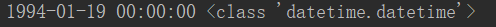datetime类型转为字符串类型
import datetime
time = datetime.datetime.strftime(datetime.datetime.now(),’%Y-%m-%d %H:%M:%S’)
print(time,type(time))
输出结果：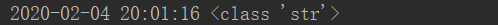时间戳转为datetime类型
10位时间戳
time = datetime.datetime.utcfromtimestamp(float(1580818427))
13位时间戳
time = datetime.datetime.utcfromtimestamp(float(1580818427123)/1000)
输出结果：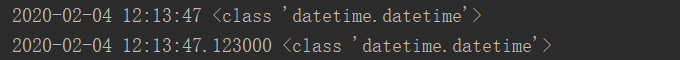datetime类型转为时间戳
time_ = datetime.datetime.timestamp(datetime.datetime.now())
输出结果：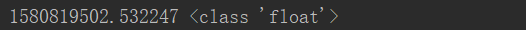字符串类型转为时间戳
time_ = ‘1994-01-19 00:00:00’
time_ = time.strptime(time_,’%Y-%m-%d %H:%M:%S’)
time = timestamp = time.mktime(time_)
输出结果：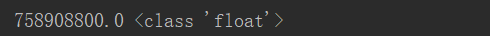获取当前时间（含日期）
time = datetime.datetime.now()
输出结果：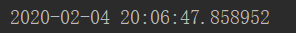获取当前时间（不含日期）
time = datetime.datetime.time(datetime.datetime.now())
输出结果：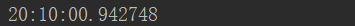获取近几天的日期
day = datetime.datetime.today() - datetime.timedelta(days=7)
for i in range(7):
print(day - datetime.timedelta(days=(-i)))
输出结果：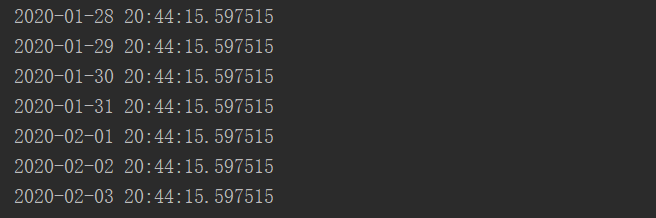python日期格式化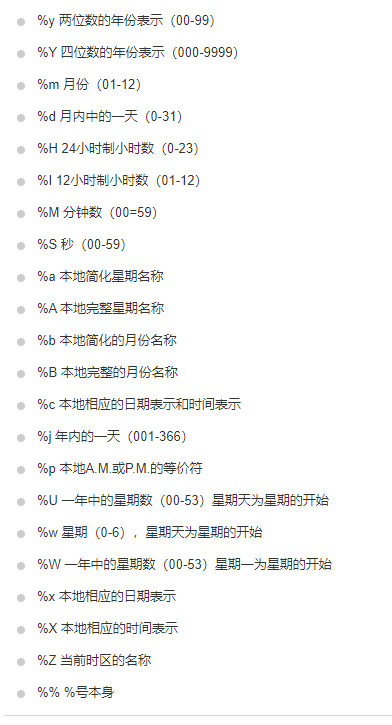展开全文• import time def get_time_to_str(str_format='%Y-%m-%d %H:%M:%S'): res = time.strftime(str_format, time.localtime(time.time())) return res def get_date_as_name(): return get_time_to_str('%Y-%m-%d'...
• strftime(format[, tuple]) -&gt; string 将指定的struct_time(默认为当前时间)，根据指定的格式化字符串...python中时间日期格式化符号： %y 两位数的年份表示（00-99） %Y 四位数的年份表示（000-9999） %m ...
• 1.Python中的time模块 1.1 time.time():输出...1.3 time.strftime():格式化成想要输出的格式： 2 time.strftime()方法参数 time.strftime里面有很多参数，可以让你能够更随意的输出自己想要的东西： 下面是ti...
• 今天小编就为大家分享一篇python日期时间转为字符串或者格式化输出的实例，具有很好的参考价值，希望对大家有所帮助。一起跟随小编过来看看吧
• 主要介绍了python日期和时间格式化输出的方法,实例总结了Python常见的日期与事件操作技巧,非常具有实用价值,需要的朋友可以参考下
• 格式化日期，需要先导入time包： import time 格式化时间使用time.strftime()方法，该方法的接口文档如下： def strftime(format, p_tuple=None): # real signature unknown; restored from __doc__ """ ...time
• Python格式化日期时间的函数为datetime.datetime.strftime()；由字符串转为日期型的函数为：datetime.datetime.strptime()，两个函数都涉及日期时间的格式化字符串，列举如下： %a Abbreviated weekday name %A ...
• 1. 日期输出格式化 所有日期、时间的api都在datetime模块内。 1. datetime => string now = datetime.datetime.now() now.strftime('%Y-%m-%d %H:%M:%S') #输出2012-03-05 16:26:23.870105 ...
• import time print (time.strftime('%Y-%m-%d %H:%M:%S',time.localtime(time.time()))) # 暂停一秒 time.sleep(2) print (time.strftime('%Y-%m-%d %H:%M:%S',time.localtime(time....输出: 2019-05-29 16...
• 本文实例总结了python日期和时间格式化输出的方法。分享给大家供大家参考。具体分析如下：python格式化日期时间的函数为datetime.datetime.strftime()；由字符串转为日期型的函数为：datetime.datetime.strptime()...
• 本文实例总结了python日期和时间格式化输出的方法。分享给大家供大家参考。具体分析如下： python格式化日期时间的函数为datetime.datetime.strftime()；由字符串转为日期型的函数为：datetime.datetime.strptime...
• 文章目录一、使用 print() 函数二、使用 str.format() 方法三、详解 format() 函数运用一：格式转换运用...在 Python 中，print() 函数支持格式化输出，与 C 语言的 printf 类似。 1. 格式化输出字符串和整数 【示例1】字符串 format
• # 获取日期： importdatetime #调用事件模块 today =datetime.date.today() #获取今天日期 deltadays =datetime.timedelta(days=1) #确定日期差额，如前天 days=2 yesterday =today -deltadays # 获取差额日期，...
• python格式化日期时间的函数为datetime.datetime.strftime()；由字符串转为日期型的函数为：datetime.datetime.strptime()，两个函数都涉及日期时间的格式化字符串，这里提供详细的代码详细演示了每一个参数的使用...
• 第一种： % 单个使用格式：“%s” % “test” 多个使用格式：“年份：%d，月份：%d，...Python 字符串格式化符号： 符号 描述 %c 格式化字符及其ASCII码 %s 格式化字符串 %d 格式化整数 %u 格式化......

# python日期格式化输出python 订阅